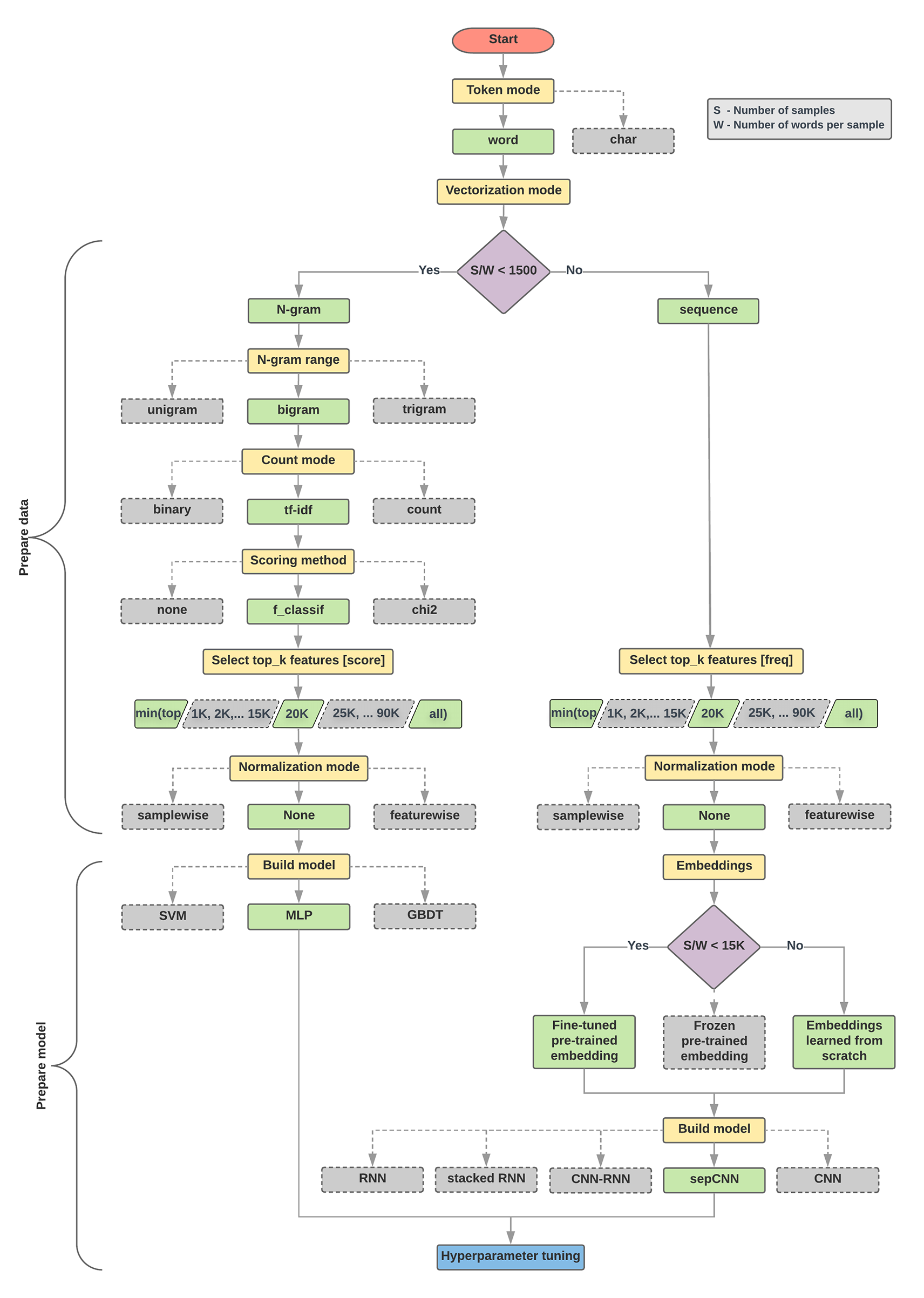# 第 2.5 步：选择模型

## 数据准备和模型构建算法

```1. Calculate the number of samples/number of words per sample ratio.
2. If this ratio is less than 1500, tokenize the text as n-grams and use a
simple multi-layer perceptron (MLP) model to classify them (left branch in the
flowchart below):
a. Split the samples into word n-grams; convert the n-grams into vectors.
b. Score the importance of the vectors and then select the top 20K using the scores.
c. Build an MLP model.
3. If the ratio is greater than 1500, tokenize the text as sequences and use a
sepCNN model to classify them (right branch in the flowchart below):
a. Split the samples into words; select the top 20K words based on their frequency.
b. Convert the samples into word sequence vectors.
c. If the original number of samples/number of words per sample ratio is less
than 15K, using a fine-tuned pre-trained embedding with the sepCNN
model will likely provide the best results.
4. Measure the model performance with different hyperparameter values to find
the best model configuration for the dataset.
```1. 我们应该使用哪种学习算法或模型？

2. 我们应该如何准备数据，以高效地了解文本和标签之间的关系？

[]
[]